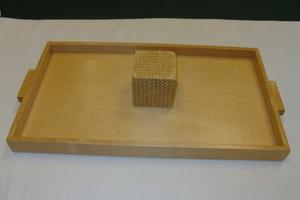# The Exchange Tray

The Exchange Tray
Math - Introduction to the Decimal System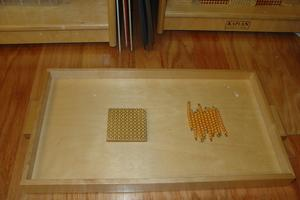LevelPrimary
Age4 - 5
9 ten bars
9 hundred squares
tray

This activity teaches the child that 10 units are the same as 1 ten, that 10 tens are the same as 1 hundred, and that 10 hundreds are the same as 1 thousand.

## Presentation

1. Prepare a tray with 10 unit beads, 9 ten bars, and 9 hundred squares.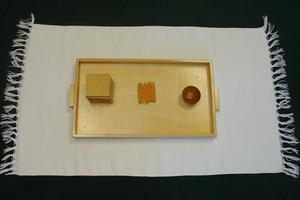2. Lay the beads out on a mat as shown.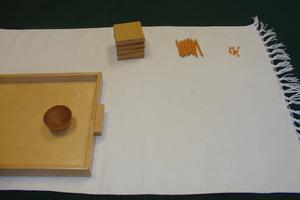3. Count the unit beads, moving them down as you do (or moving them into a small bowl). "1 unit, 2 units, 3 units..."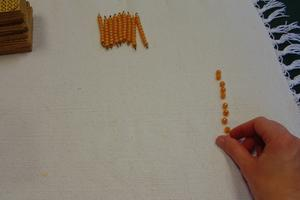4. "10 units is the same as 1 ten." Have the child go to the bank and exchange the 10 unit beads for 1 ten.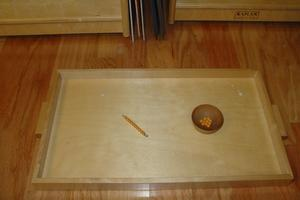5. Bring the ten bar to the mat.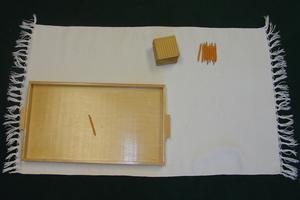6. Place it with the other ten bars.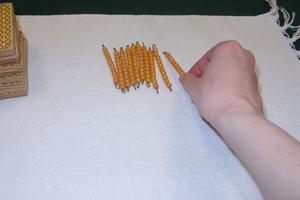7. Count the ten bars: "1 ten, 2 tens, 3 tens..."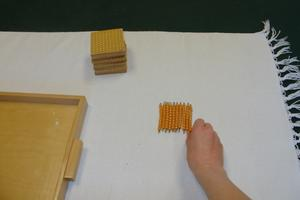8. "10 tens is the same as 1 hundred." Have the child go to the bank and exchange the 10 ten bars for 1 hundred square.9. Bring the hundred square to the mat. Place it with the other hundred squares.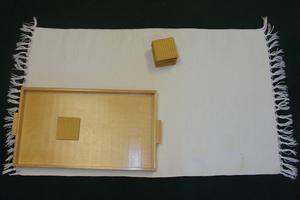10. Count the hundred squares: "1 hundred, 2 hundreds, 3 hundreds..."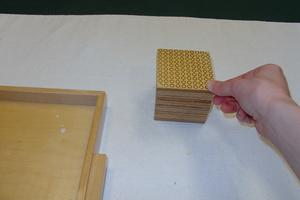11. "10 hundreds is the same as 1 thousand." Have the child go to the bank and exchange the 10 hundred squares for 1 thousand.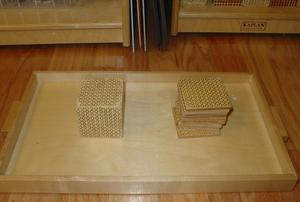12. Bring the thousand cube to the mat. "One thousand. Did you know that we had one thousand beads on the tray?"